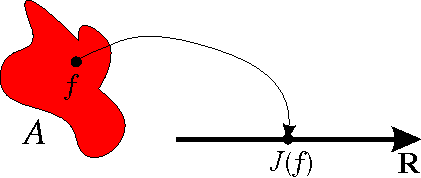2. Functionals - extremals.

A (one variable) function maps real numbers on real numbers. We now introduce the notion of functional. A functional maps functions on real numbers. Consider a real valued functionalwhere A is a set of admissible functions.We are interested in solving what is called the fundamental problem of calculus of variations: Given a functional J and a set A of admissible functions, find the functions in A that gives an extreme value to J.

Remark: The functions that give extreme values to J are called extremals. The problem of finding extremals for a functional J over a set A is called a variational problem.